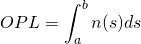# Object distance

Distance between the object side principal point and the object.
For larger distances, this is about the working distance

# optical axis

The symmetry axis of a lens or a lens system.

# Optical Density

Optical density is a measure used for filters to describe how much light of a blocked wavelength still passes the filter

Abbreviation: ODn

the optical density is given by the formula:
ODn = 10^-n = 1/10^n
OD0 = 10^-0 = 1/10^0 = 1 = 100% light passes
OD1 = 10^-1 = 1/10^1 = 0.1 = 10% light passes
OD2 = 10^-2 = 1/10^2 = 0.01 = 1% light passes
OD3 = 10^-3 = 1/10^3 = 0.001 = 0.1% light passes
OD4 = 10^-4 = 1/10^4 = 0.0001 = 0.01% light passes
The optical density is an input value, demanded by the Application.
OD2 equals 1 percent.
For a camera of 256 intensity levels this equals 2.6 intesity values. This is not much stronger than the sensor noise.

OD3 entspricht 0.1 Prozent.
For a camera of 256 intensity levels this equals 0.26 intesity values. This is less than the sensor noise.

The higher the number the better the blocking.
The higher the number the more expensive the filter.
Typical values for comemrcial filters are OD2,
higher quality filters have OD3
Filters ilters for outdoor use might need OD4 or higher to block too intensive sunlight.
For a + b = c we get ODa + ODb = ODc
so you can generater an OD6 filter by two OD3 filters. However, the optics should be prepared for the thicker glass then.

# optical magnificationMay the width of an object be 1.6mm and the sensor width = 4.8mm
ThenMay the width of an object be 36mm and the sensor width = 3.6mm
Then# Optical Path Length

The optical path length is proportional to the time light needs to travel between two pointsIn a homogenous medium with refractuion index n and physical point distance d this formula simplifies toIn glass with the index of refraction of 1.5 for some wavelength for a physical point distance of d=10mm the optical path lengthAs glass has a different index of refraction for different wavelengths, the OPL is different for each wavelength
Some OPLs for glass type BK7 and a physical point distance of d=10mm
[table caption=”sample OPLs for BK7″ width=”400″ colwidth=”100|100|100|100″ colalign=”left|left|left”]
wavelength,index of refraction,phys. distance, OPL
400nm,1.5308,10mm,15.308mm,
500nm,1.5214,10mm,15.214mm,
600nm,1.5163,10mm,15.163mm,
850nm,1.5098,10mm,15.098mm,
940nm,1.5084,10mm,15.084mm
[/table]
In paraxial imaging system all of the light rays connecting a source point a to it’s image point have equal OLPs

# orthographic

orthographic lenses are a type of fisheye lenses

orthographic lenses are also called” hemispherical“or “orthographisch
orthographic lenses use an image mapping function of typemaintains planar illuminance
[table caption=”orthographic angles” width=”500″ colwidth=”50|50|50|50″ colalign=”center|center|center|center”]
weak,medium,strong,max
66,90,120,180
[/table]An orthographic / hemispherical fisheye, uses a parallel projection of a hemisphere onto a plane. The resulting image will obviously be circular. The widest view angle is 180 degrees. At +/-90 degrees extreme distortion will occur. Due to this heavy distortion it’s less often used than f-Theta / angular fisheye.

see:
fisheye types